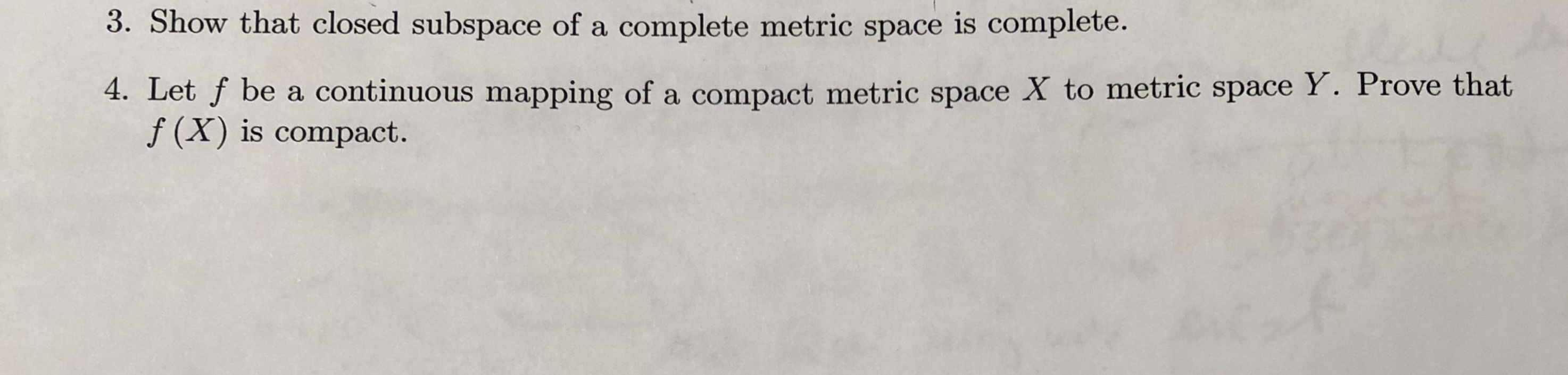3. Show that closed subspace of a complete metric space is complete.4. Let f be a continuous mapping of a compact metric space X to metric space Y. Prove thatf (X) is compact.A 1S

Questionhelp_outlineImage Transcriptionclose3. Show that closed subspace of a complete metric space is complete. 4. Let f be a continuous mapping of a compact metric space X to metric space Y. Prove that f (X) is compact. A 1S fullscreen
Step 1

As per norms, the first question is answered: To prove that a closed subspace Y of a complete metric space X is complete.

Step 2

A metric space X is complete if all Cauchy sequences in X converge in X. A subspace Y of X is closed if it containes all the limit points of sequences in Y. We need to show that Y is complete in itself (in the induced topology form X); in other words, every Cauchy sequence in Y converges in Y.

Step 3

Want to see the full answer?

See Solution

Want to see this answer and more?

Our solutions are written by experts, many with advanced degrees, and available 24/7

See Solution
Tagged in

Math